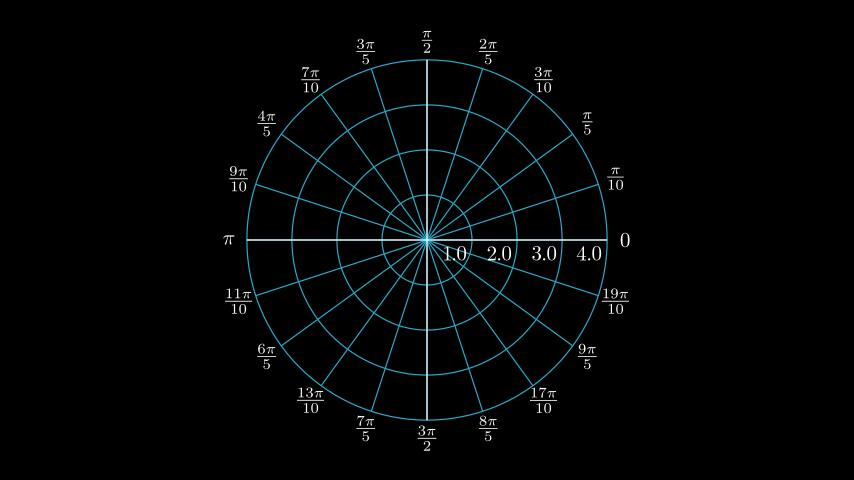# PolarPlane#

Qualified name: manim.mobject.graphing.coordinate\_systems.PolarPlane

Bases: Axes

Creates a polar plane with background lines.

Parameters
• azimuth_step (float | None) –

The number of divisions in the azimuth (also known as the angular coordinate or polar angle). If None is specified then it will use the default specified by azimuth_units:

• "PI radians" or "TAU radians": 20

• "degrees": 36

• "gradians": 40

• None: 1

A non-integer value will result in a partial division at the end of the circle.

• size (float | None) – The diameter of the plane.

• azimuth_units (str | None) –

Specifies a default labelling system for the azimuth. Choices are:

• "PI radians": Fractional labels in the interval $$\left[0, 2\pi\right]$$ with $$\pi$$ as a constant.

• "TAU radians": Fractional labels in the interval $$\left[0, \tau\right]$$ (where $$\tau = 2\pi$$) with $$\tau$$ as a constant.

• "degrees": Decimal labels in the interval $$\left[0, 360\right]$$ with a degree ($$^{\circ}$$) symbol.

• "gradians": Decimal labels in the interval $$\left[0, 400\right]$$ with a superscript “g” ($$^{g}$$).

• None: Decimal labels in the interval $$\left[0, 1\right]$$.

• azimuth_compact_fraction (bool) – If the azimuth_units choice has fractional labels, choose whether to combine the constant in a compact form $$\tfrac{xu}{y}$$ as opposed to $$\tfrac{x}{y}u$$, where $$u$$ is the constant.

• azimuth_offset (float) – The angle offset of the azimuth, expressed in radians.

• azimuth_direction (str) –

The direction of the azimuth.

• "CW": Clockwise.

• "CCW": Anti-clockwise.

• azimuth_label_buff (float) – The buffer for the azimuth labels.

• azimuth_label_font_size (float) – The font size of the azimuth labels.

• radius_config (dict | None) – The axis config for the radius.

• background_line_style (dict | None) –

• faded_line_style (dict | None) –

• make_smooth_after_applying_functions (bool) –

Examples

Example: PolarPlaneExamplefrom manim import *

class PolarPlaneExample(Scene):
def construct(self):
polarplane_pi = PolarPlane(
size=6,
azimuth_label_font_size=33.6,


References: PolarPlane

Methods

 add_coordinates Adds the coordinates. get_axes Gets the axes. get_coordinate_labels Gets labels for the coordinates get_radian_label get_vector prepare_for_nonlinear_transform

Attributes

 animate Used to animate the application of any method of self. animation_overrides color depth The depth of the mobject. fill_color If there are multiple colors (for gradient) this returns the first one height The height of the mobject. n_points_per_curve sheen_factor stroke_color width The width of the mobject.

Parameters
• r_values (Iterable[float] | None) – Iterable of values along the radius, by default None.

• a_values (Iterable[float] | None) – Iterable of values along the azimuth, by default None.

get_axes()[source]#

Gets the axes.

Returns

A pair of axes.

Return type

VGroup

get_coordinate_labels(r_values=None, a_values=None, **kwargs)[source]#

Gets labels for the coordinates

Parameters
• r_values (Iterable[float] | None) – Iterable of values along the radius, by default None.

• a_values (Iterable[float] | None) – Iterable of values along the azimuth, by default None.

Returns

Labels for the radius and azimuth values.

Return type

VDict